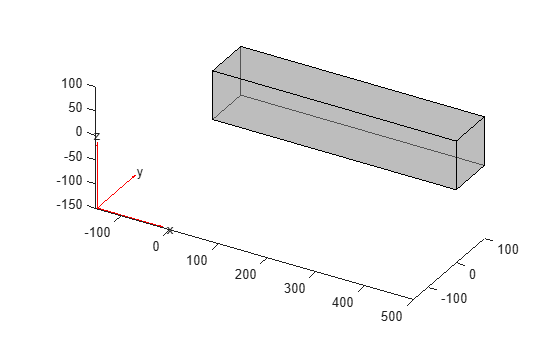Documentation

# structuralDamping

Specify damping parameters for transient or frequency response structural model

## Syntax

``structuralDamping(structuralmodel,'proportional','Alpha',a,'Beta',b)``
``ab = structuralDamping(___)``

## Description

example

````structuralDamping(structuralmodel,'proportional','Alpha',a,'Beta',b)` specifies proportional (Rayleigh) damping parameters `a` and `b` for `structuralmodel`. The second argument must be `'proportional'`.For a frequency response model with damping, the results are complex. Use functions such as `abs` and `angle` to obtain real-valued results such as magnitude and phase.```
````ab = structuralDamping(___)` returns the damping parameters object, using the previous syntax.```

## Examples

collapse all

Specify proportional (Rayleigh) damping parameters for a beam.

Create a transient structural model.

` structuralModel = createpde('structural','transient-solid');`

Import and plot the geometry.

``` gm = importGeometry(structuralModel,'SquareBeam.STL'); pdegplot(structuralModel,'FaceAlpha',0.5)```Specify the Young's modulus, Poisson's ratio, and mass density.

```structuralProperties(structuralModel,'YoungsModulus',210E9,... 'PoissonsRatio',0.3,... 'MassDensity',7800);```

Specify the Rayleigh damping parameters.

`structuralDamping(structuralModel,'proportional','Alpha',10,'Beta',2)`
```ans = StructuralDampingAssignment with properties: RegionType: 'Cell' RegionID: 1 DampingModel: 'proportional' Alpha: 10 Beta: 2 ```

## Input Arguments

collapse all

Transient or frequency response structural model, specified as a `StructuralModel` object. The model contains the geometry, mesh, structural properties of the material, body loads, boundary loads, boundary conditions, and initial conditions.

Example: ```structuralmodel = createpde('structural','transient-solid')```

Mass proportional damping, specified as a real number.

Data Types: `double`

Stiffness proportional damping, specified as a real number.

Data Types: `double`

## Output Arguments

collapse all

Handle to damping parameters, returned as a `StructuralDampingAssignment` object.